Courses

# If x, y, z are non-zero numbers and x + xy2 = z3, then which of the following is NOT equal to 1?a)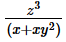b)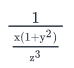c)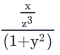d)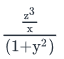e)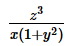Correct answer is option 'C'. Can you explain this answer? Related Test: Test: Simplifying Expression

## GMAT Question

By DEEPAK PRAJAPAT · Apr 05, 2020 ·GMAT
Harmanjot Singh answered Apr 07, 2020
Given equation is
x+xy2 = z3
This can be rewritten as:
x(1+y2) = z …………….. (I)
We need to find the option statement whose value is not equal to 1.
Now let us look at each option.
Option A:This is same as…………….. Expression A
From (I), we know that the numerator and denominator of Expression A are equal.
Therefore the value of Expression A is 1.
Option B:This can be rewritten as: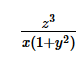We already saw in the previous option that this is equal to 1.
Option C:This can be rewritten as: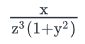From I, we can rewrite this as: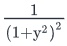………….. Expression C
It is given that y is a non-zero number. Therefore, the value of  (1+y2) is always greater than 1.
So, Expression C cannot be equal to 1.
Option D:This can be rewritten as: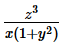From options A and B, we already know that this expression is equal to 1.
Option E: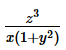We already know that this expression is equal to 1.